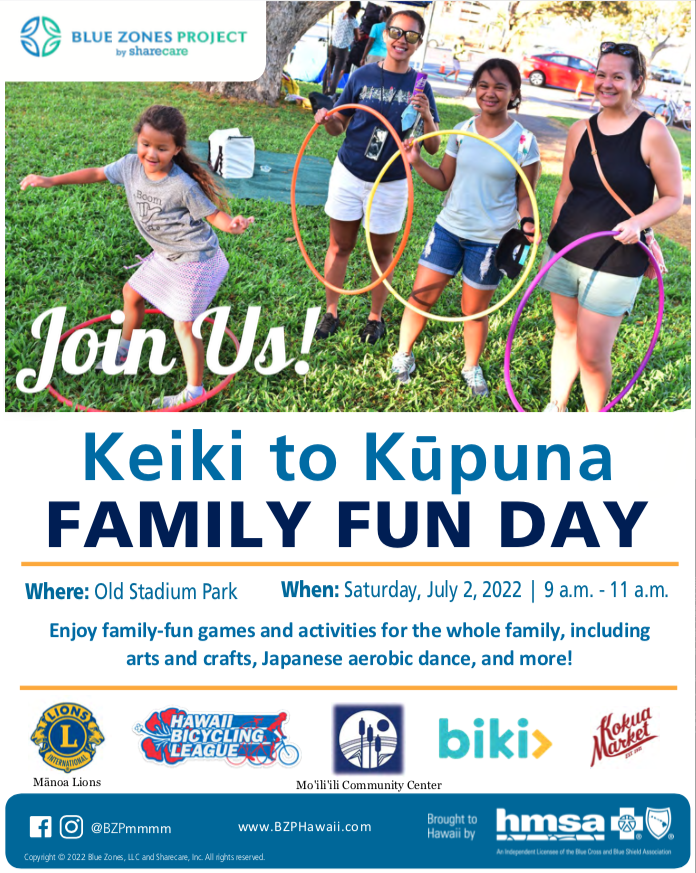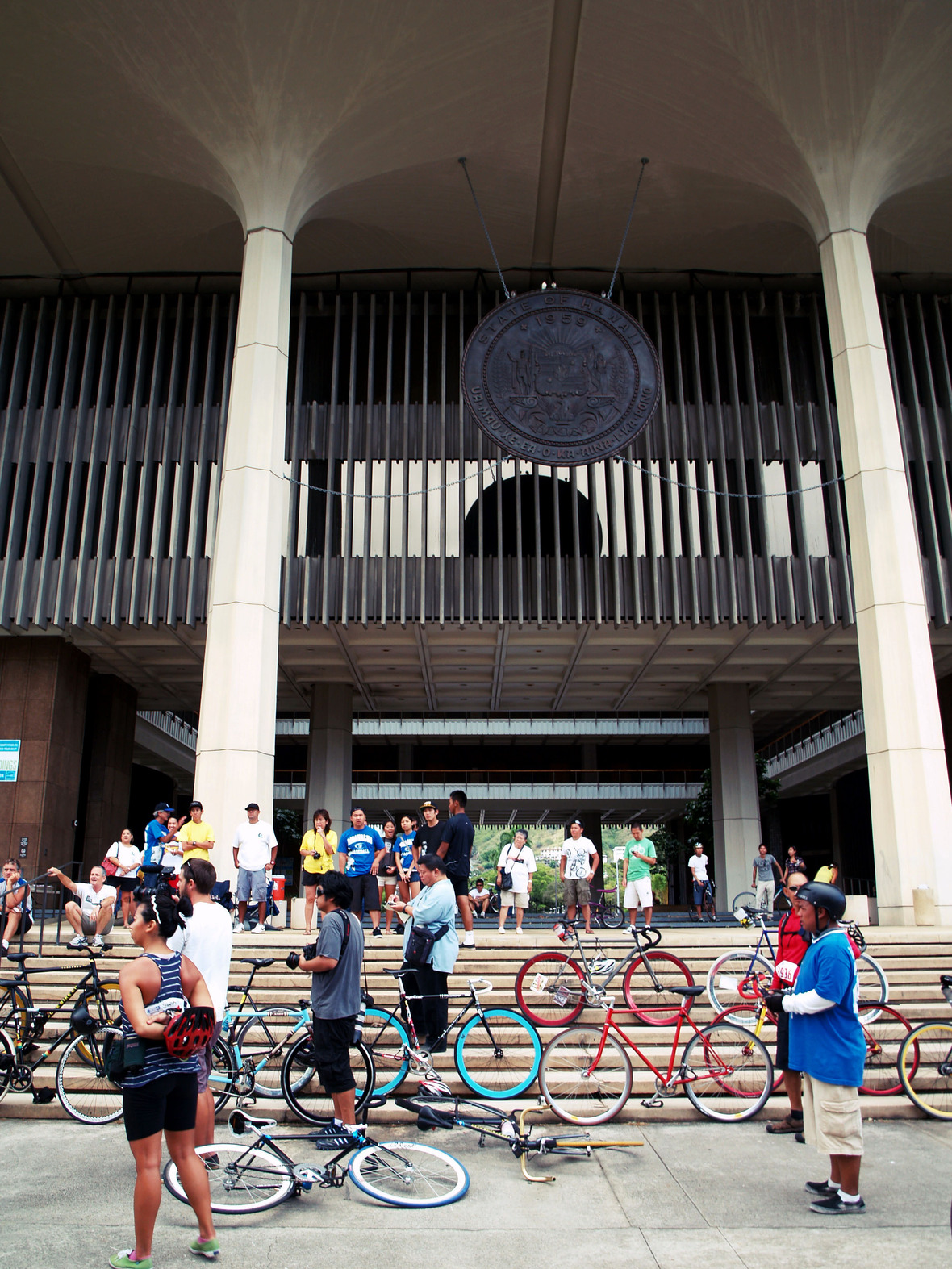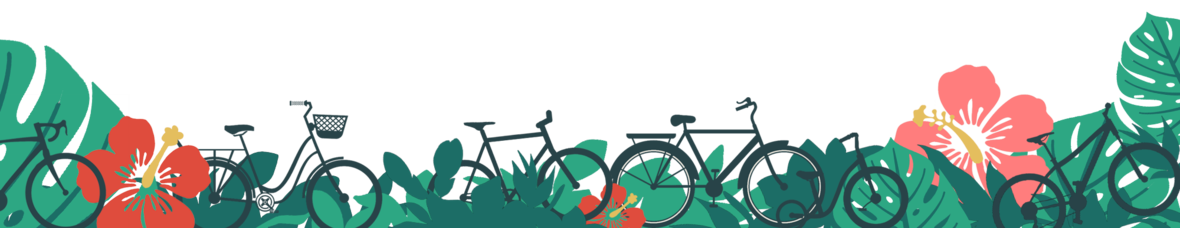## This year we'll be holding Bike Month in the month of July

Your friends at the Hawaii Bicycling League have been hard at work planning a BUNCH of BIKE-TASTIC events throughout the entire month to encourage people to get pedaling and to reward those already riding. So tell your co-workers, grab your biking buddies, and join us as we celebrate all of July in super cyclin' style!

## Find out all the details at:

 table div table+table+table+table+table+table div table{width:100%;padding:0}table div table+table+table+table+table+table div table img{width:96.23%;padding:0;float:none}table div table+table+table+table+table+table div table td{width:100%;padding:0 1.88% 18px}/* styles */table div table+table+table+table+table+table+table div table td,table.module-6{width:100%;padding:0}table div table+table+table+table+table+table+table div table{width:100%;float:none;margin-left:auto;margin-right:auto;padding:0}table div table+table+table+table+table+table+table div table a{border:0 none;text-decoration:none}table div table+table+table+table+table+table+table div table img{width:100%!important;border:0 none;text-decoration:none}/* styles */

# WHEN: Every Tuesday & Friday in July!

## WHERE: @Various locations

WHAT: HBL, Bikeshare Hawaii, and the C&C Department of Transportation Services are hosting Bike Month Energizer Stations where you can get rewarded for biking! Mahalo to 7-Eleven Hawaii, Planet Fitness, and Starbucks at SALT for providing ‘ono treats & strong coffee.

 /* styles */ ⌶ 7/05 (Tue) ◈ 3:30 – 6pm @ S. King St. + Ke‘eaumoku St. ⋯ Biki Energizer Station Sponsored by 7-Eleven Hawaii ⌶ 7/08 (Fri) ◈ 7 – 9am @Fasi Municipal Building ⋯ DTS Bike Month Station ⌶ 7/12 (Tue) ◈ 3:30 – 6pm @Biki Stop #244 (Ala Moana Beach Park) ⋯ Biki Energizer Station Sponsored by Project Footprint ⌶ 7/15 (Fri) ◈ 11am – 1pm @Fasi Municipal Building ⋯ DTS Bike Month Station ⌶ 7/19 (Tue) ◈ 3:30 – 6pm @Biki Stop #228 (Ala Moana) ⋯ Biki Energizer Station Sponsored by Planet Fitness ⌶ 7/22 (Fri) ◈ 7 – 9am @Civic Center Bike Path + Punchbowl St. ⋯ HBL Bike to Work Day Energizer Station Sponsored by Starbucks SALT ⌶ 7/26 (Tue) ◈ 3:30 – 6pm @TBA ⋯ Biki Energizer Station ⌶ 7/29 (Fri) ◈ 3 – 5pm @Fasi Municipal Building ⋯ DTS Bike Month Station

## For Energizer Station details - like what kind of goodies are being given out at each one & their specific locations - go to: HBL.org/BikeMonth!

 table div table+table+table+table+table+table+table+table+table+table+table+table div table td,table.module-11{width:100%;padding:0}table div table+table+table+table+table+table+table+table+table+table+table+table div table{width:100%;float:none;margin-left:auto;margin-right:auto;padding:0}table div table+table+table+table+table+table+table+table+table+table+table+table div table a{border:0 none;text-decoration:none}table div table+table+table+table+table+table+table+table+table+table+table+table div table img{width:100%!important;border:0 none;text-decoration:none}/* styles */Hosted by Blue Zones Project

# WHEN: July 2nd (SAT) ◈ 9 - 11am

WHAT: Enjoy family-fun games and activities for the whole family, including arts and crafts, Japanese aerobic dance, and more! This Blue Zones Project event is free and open to the public.

 table div table+table+table+table+table+table+table+table+table+table+table+table+table+table div table{width:100%;padding:0}table div table+table+table+table+table+table+table+table+table+table+table+table+table+table div table img{width:96.23%;padding:0;float:none}table div table+table+table+table+table+table+table+table+table+table+table+table+table+table div table td{width:100%;padding:0 1.88% 18px}/* styles */table div table+table+table+table+table+table+table+table+table+table+table+table+table+table+table div table td,table.module-14{width:100%;padding:0}table div table+table+table+table+table+table+table+table+table+table+table+table+table+table+table div table{width:100%;float:none;margin-left:auto;margin-right:auto;padding:0}table div table+table+table+table+table+table+table+table+table+table+table+table+table+table+table div table a{border:0 none;text-decoration:none}table div table+table+table+table+table+table+table+table+table+table+table+table+table+table+table div table img{width:100%!important;border:0 none;text-decoration:none}/* styles */

# WHEN: July 9th (SAT)

WHERE: Check out HBL.org/BikeMonth for the party location!

WHAT: Time to bust out your aloha shirts for this casual ride that will incorporate a stop for shave ice. Tacky or Terrific; Fugly or Fantastic... all Aloha Shirts are welcome here. We hope to see a gamut of floral prints for this urban Honolulu social cruise!

 table div table+table+table+table+table+table+table+table+table+table+table+table+table+table+table+table+table div table{width:100%;padding:0}table div table+table+table+table+table+table+table+table+table+table+table+table+table+table+table+table+table div table img{width:96.23%;padding:0;float:none}table div table+table+table+table+table+table+table+table+table+table+table+table+table+table+table+table+table div table td{width:100%;padding:0 1.88% 18px}/* styles */# 7/11 FREE BIKI RIDES DAY

## WHEN: July 11th (MON) ◈ 12:01am - 11:59pm

WHERE: Anywhere!
WHAT: Free Biki rides all day long, sponsored by 7-Eleven Hawaii. Use code 711 at any Biki Stop or on the PBSC App to redeem one free 30 minute ride.

 table div table+table+table+table+table+table+table+table+table+table+table+table+table+table+table+table+table+table+table div table{width:100%;padding:0}table div table+table+table+table+table+table+table+table+table+table+table+table+table+table+table+table+table+table+table div table img{width:96.23%;padding:0;float:none}table div table+table+table+table+table+table+table+table+table+table+table+table+table+table+table+table+table+table+table div table td{width:100%;padding:0 1.88% 18px}/* styles */table div table+table+table+table+table+table+table+table+table+table+table+table+table+table+table+table+table+table+table+table div table td,table.module-19{width:100%;padding:0}table div table+table+table+table+table+table+table+table+table+table+table+table+table+table+table+table+table+table+table+table div table{width:100%;float:none;margin-left:auto;margin-right:auto;padding:0}table div table+table+table+table+table+table+table+table+table+table+table+table+table+table+table+table+table+table+table+table div table a{border:0 none;text-decoration:none}table div table+table+table+table+table+table+table+table+table+table+table+table+table+table+table+table+table+table+table+table div table img{width:100%!important;border:0 none;text-decoration:none}/* styles */Discover new things in this cycling scavenger hunt - and win prizes!

# WHEN: July 16th (SAT) ◈ 8am – 1pm

## WHERE: Start @Kaka‘ako, Finish @State Capitol

WHAT: Zach's Scavenger Hunt in Paradise is open to riders of all ages and sends cyclists out on a cycling scavenger hunt where they'll collect checkpoints in the hunt to rack up the most points to win awesome prizes! There is no set route as this is designed so that you make your own adventure, but entrants can expect to ride as short as 2-miles or as long as 30 miles. We will finish at the State Capitol where there will be a rally for safe streets and lunch (provided for all)!

 table div table+table+table+table+table+table+table+table+table+table+table+table+table+table+table+table+table+table+table+table+table+table div table{width:100%;padding:0}table div table+table+table+table+table+table+table+table+table+table+table+table+table+table+table+table+table+table+table+table+table+table div table img{width:96.23%;padding:0;float:none}table div table+table+table+table+table+table+table+table+table+table+table+table+table+table+table+table+table+table+table+table+table+table div table td{width:100%;padding:0 1.88% 18px}/* styles */table div table+table+table+table+table+table+table+table+table+table+table+table+table+table+table+table+table+table+table+table+table+table+table div table td,table.module-22{width:100%;padding:0}table div table+table+table+table+table+table+table+table+table+table+table+table+table+table+table+table+table+table+table+table+table+table+table div table{width:100%;float:none;margin-left:auto;margin-right:auto;padding:0}table div table+table+table+table+table+table+table+table+table+table+table+table+table+table+table+table+table+table+table+table+table+table+table div table a{border:0 none;text-decoration:none}table div table+table+table+table+table+table+table+table+table+table+table+table+table+table+table+table+table+table+table+table+table+table+table div table img{width:100%!important;border:0 none;text-decoration:none}/* styles */

# WHEN: July 27th (WED) ◈ 5pm

WHERE: @Check out HBL.org/BikeMonth for the party location!
WHAT: Join us on Wednesday, 7/27 at 5pm for Bike Month Pau Hana! Celebrate bicycles and connect with friends. Location & details coming soon!

 table div table+table+table+table+table+table+table+table+table+table+table+table+table+table+table+table+table+table+table+table+table+table+table+table+table div table{width:100%;padding:0}table div table+table+table+table+table+table+table+table+table+table+table+table+table+table+table+table+table+table+table+table+table+table+table+table+table div table img{width:96.23%;padding:0;float:none}table div table+table+table+table+table+table+table+table+table+table+table+table+table+table+table+table+table+table+table+table+table+table+table+table+table div table td{width:100%;padding:0 1.88% 18px}/* styles */table div table+table+table+table+table+table+table+table+table+table+table+table+table+table+table+table+table+table+table+table+table+table+table+table+table+table div table td,table.module-25{width:100%;padding:0}table div table+table+table+table+table+table+table+table+table+table+table+table+table+table+table+table+table+table+table+table+table+table+table+table+table+table div table{width:100%;float:none;margin-left:auto;margin-right:auto;padding:0}table div table+table+table+table+table+table+table+table+table+table+table+table+table+table+table+table+table+table+table+table+table+table+table+table+table+table div table a{border:0 none;text-decoration:none}table div table+table+table+table+table+table+table+table+table+table+table+table+table+table+table+table+table+table+table+table+table+table+table+table+table+table div table img{width:100%!important;border:0 none;text-decoration:none}/* styles */

# WHEN: July 29th (FRI) & July 30th (SAT) ◈ 4pm – 10pm

## WHERE: Waikīkī Shell

WHAT: Bike to the concert! HBL will be hosting the bike valet program at Jack Johnson’s show at the Waikīkī Shell on both evenings. Bike to the show, park at our bike valet station and enter to win great prizes including a custom Jack Johnson Solé Bicycle!

 table div table+table+table+table+table+table+table+table+table+table+table+table+table+table+table+table+table+table+table+table+table+table+table+table+table+table+table+table div table{width:100%;padding:0}table div table+table+table+table+table+table+table+table+table+table+table+table+table+table+table+table+table+table+table+table+table+table+table+table+table+table+table+table div table img{width:96.23%;padding:0;float:none}table div table+table+table+table+table+table+table+table+table+table+table+table+table+table+table+table+table+table+table+table+table+table+table+table+table+table+table+table div table td{width:100%;padding:0 1.88% 18px}/* styles */table div table+table+table+table+table+table+table+table+table+table+table+table+table+table+table+table+table+table+table+table+table+table+table+table+table+table+table+table+table div table td,table.module-28{width:100%;padding:0}table div table+table+table+table+table+table+table+table+table+table+table+table+table+table+table+table+table+table+table+table+table+table+table+table+table+table+table+table+table div table{width:100%;float:none;margin-left:auto;margin-right:auto;padding:0}table div table+table+table+table+table+table+table+table+table+table+table+table+table+table+table+table+table+table+table+table+table+table+table+table+table+table+table+table+table div table a{border:0 none;text-decoration:none}table div table+table+table+table+table+table+table+table+table+table+table+table+table+table+table+table+table+table+table+table+table+table+table+table+table+table+table+table+table div table img{width:100%!important;border:0 none;text-decoration:none}/* styles */

# WHEN: July 31st (SUN) ◈ 10am – 2pm

## WHERE: @The Barn at SALT Kaka‘ako

WHAT: Biki and HBL will be performing free helmet fittings and distributing over 400 helmets to members of the community, sponsored by Central Pacific Bank.

 table div table+table+table+table+table+table+table+table+table+table+table+table+table+table+table+table+table+table+table+table+table+table+table+table+table+table+table+table+table+table+table div table{width:100%;padding:0}table div table+table+table+table+table+table+table+table+table+table+table+table+table+table+table+table+table+table+table+table+table+table+table+table+table+table+table+table+table+table+table div table img{width:96.23%;padding:0;float:none}table div table+table+table+table+table+table+table+table+table+table+table+table+table+table+table+table+table+table+table+table+table+table+table+table+table+table+table+table+table+table+table div table td{width:100%;padding:0 1.88% 18px}/* styles */# Get Your Limited Edition 2022 Bike Month Tee!

Stop by any one of HBL's Bike Month events to score a LIMITED EDITION Bike Month shirt of your own! We'll have an assortment of different types of shirts & colours to pick from to sit your specific style... so get them before your favourite one is gone!

\$20 for Non-Members - join today!
Half-off for HBL Members (just 10 bucks)!

 table div table+table+table+table+table+table+table+table+table+table+table+table+table+table+table+table+table+table+table+table+table+table+table+table+table+table+table+table+table+table+table+table+table div table{width:100%;padding:0}table div table+table+table+table+table+table+table+table+table+table+table+table+table+table+table+table+table+table+table+table+table+table+table+table+table+table+table+table+table+table+table+table+table div table img{width:96.23%;padding:0;float:none}table div table+table+table+table+table+table+table+table+table+table+table+table+table+table+table+table+table+table+table+table+table+table+table+table+table+table+table+table+table+table+table+table+table div table td{width:100%;padding:0 1.88% 18px}/* styles */# Like Biking?

Support HBL's efforts in making Hawai‘i the pedaling paradise it deserves to be.

## Here's how YOU can make a difference!

1. Encourage a friend, coworker, family member to ride! Show them a comfortable bike route for their riding abilities, have 'em get confident on the bike by bringing them out to one of HBL's fun, informative, and free(!) hands-on cycling workshops, and welcome them into the cycling ʻohana with one of HBL's many wonderful group rides!

2. Help at one (or more!) of the Bike Month events. It will only take a couple hours of your day and you are sure to go home feeling pumped AND very appreciated! Click here to see where you could help.

3. Make a donation to HBL, the local nonprofit behind the scenes organizing these events and doing work in advocacy and education to make Hawaiʻi a bicycle paradise at HBL.org/Support. All donations are tax-deductible. Support HBL during Bike Month! The Johnson Ohana Foundation will be matching donations, up to a total \$2500. Double your contribution! Plus, be entered to win a pair of tickets to the Jack Johnson Concert on Friday, July 29th! More details coming soon.

 table.module-34{width:47.36%;padding:0}table div table+table+table+table+table+table+table+table+table+table+table+table+table+table+table+table+table+table+table+table+table+table+table+table+table+table+table+table+table+table+table+table+table+table+table div table{width:47.36%;float:none;margin-left:auto;margin-right:auto;padding:0}table div table+table+table+table+table+table+table+table+table+table+table+table+table+table+table+table+table+table+table+table+table+table+table+table+table+table+table+table+table+table+table+table+table+table+table div table a{border:0 none;text-decoration:none}table div table+table+table+table+table+table+table+table+table+table+table+table+table+table+table+table+table+table+table+table+table+table+table+table+table+table+table+table+table+table+table+table+table+table+table div table img{width:100%!important;border:0 none;text-decoration:none}table div table+table+table+table+table+table+table+table+table+table+table+table+table+table+table+table+table+table+table+table+table+table+table+table+table+table+table+table+table+table+table+table+table+table+table div table td{width:100%;padding:0}/* styles */

# Happy Bike Month & Ride Aloha!

## ♥ HBL Staff

Travis Counsell - Executive Director
Malia k Harunaga - Director of Adult Education
Andrew Ouchi - Youth BikeEd Manager
Lauren Lee - Events & Marketing Coordinator